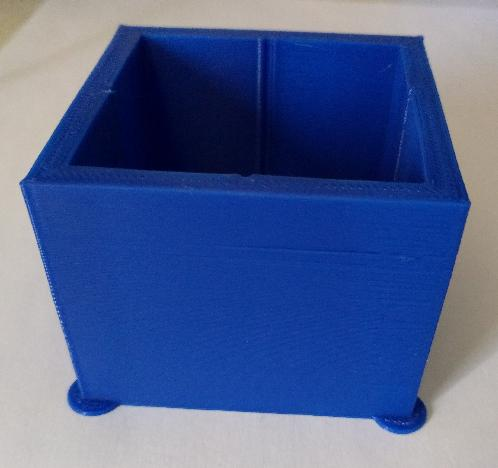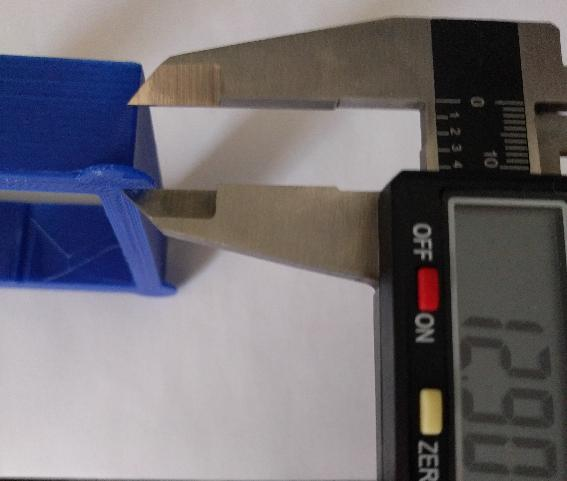Pressure advance有兩個作用 - 它可以減少非擠出移動過程中的溢料和減少轉彎時的凸起。本指南使用第二個功能（減少轉彎過程中的凸起）作為優化機制。

``````SET_VELOCITY_LIMIT SQUARE_CORNER_VELOCITY=1 ACCEL=500
``````

``````TUNING_TOWER COMMAND=SET_PRESSURE_ADVANCE PARAMETER=ADVANCE START=0 FACTOR=.005
``````

``````TUNING_TOWER COMMAND=SET_PRESSURE_ADVANCE PARAMETER=ADVANCE START=0 FACTOR=.020
``````